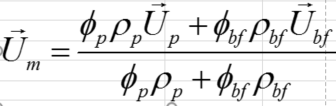## Fluids

Topics relate to Fluent, CFX, Turbogrid and more

•Juwelmojumder1
Subscriber
Hi,nI'm trying to simulate the Mixture model for the fluid flow, and DO model for the solar input. In the mixture model, I need to give properties for individual phases, including optical properties like absorption coefficients. For the single phase model condition, it's straight forward to calculate the absorption coefficients (peoples use Rayleigh/Mie theory). For the mixture model, If I give the value of individual value of phases, the results shows a higher deviation, which is lower for the single phase condition. Is there any rule/formula to calculate this optical properties or the mixture condition?nLastly, In the mixture model, what will be the phase's velocity? Do they require equal velocity, or the dissimilar velocity?nThanksnJuweln
•Rob
Ansys Employee
You need to be careful with multiphase & radiation absorption/scattering. Assuming the gas phase has zero absorption, will 2m at 25% vol fraction behave the same as 1m at 50%? nFor phase velocity, that depends entirely on the model used and what you're modelling. Mixture model shares the momentum field, Euler doesn't. n
•Juwelmojumder1
Subscriber
Hi,nThanks for your reply. nIn my case, nanoparticles (secondary phase) is dispersed in the water. Here, individual phases have their absorption coefficient. There is no information regarding the mixture properties of this value in the Fluent-help/theory guide. Do they follow the mixture rule like other thermophysical properties? nFor the velocity calculation, there are many possible options of giving the velocity-input for each phase under a constant volume fraction and known mixture velocity, according to the following equation available in Fluent theory.nThen, how I'll to provide the exact velocity inputs? Do I need to continue the above equation iteratively to find the best fitted velocity pairs so that it validate with the experimental data? n